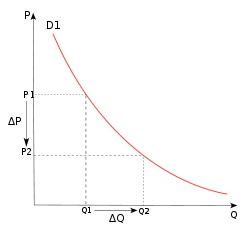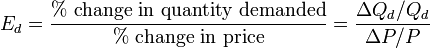• Have any questions?
• +91-9540-660064
• hahassignment@gmail.com

# Elasticity of Demand

Price Elasticity of Demand (PED or Ed) is a measure used in economics to show the responsiveness, or elasticity, of the quantity demanded of a good or service to a change in its price. More precisely, it gives the percentage change in quantity demanded in response to a one percent change in price (holding constant all the other determinants of demand, such as income). It was devised by Alfred Marshall. Price elasticities are almost always negative, although analysts tend to ignore the sign even though this can lead to ambiguity. Only goods which do not conform to the law of demand, such as Veblen and Giffen goods, have a positive PED. In general, the demand for a good is said to be inelastic (or relatively inelastic) when the PED is less than one (in absolute value): that is, changes in price have a relatively small effect on the quantity of the good demanded. The demand for a good is said to be elastic (or relatively elastic) when its PED is greater than one (in absolute value): that is, changes in price have a relatively large effect on the quantity of a good demanded. Revenue is maximised when price is set so that the PED is exactly one. The PED of a good can also be used to predict the incidence (or "burden") of a tax on that good. Various research methods are used to determine price elasticity, including test markets, analysis of historical sales data and conjoint analysis.PED is a measure of responsiveness of the quantity of a good or service demanded to changes in its price.The formula for the coefficient of price elasticity of demand for a good is:### Application of Differentiation in Economics:

Economics deals with  cost and revenue functions.
The cost functions are average cost (AC), marginal cost (MC) and marginal average cost.
The revenue functions are  total revenue R, average revenue (AR) and marginal revenue (MR)
Profit is Revenue - cost.
So every economist will try to minimize the cost and maximise the revenue to get a maximum profit.
The cost functions has two components.  They are the fixed cost and the variable  cost.
Cost(C) is a function of output x . Hence C = f(x)
Average cost (AC) = total cost / output = C/x
Marginal cost is dC/dx
Marginal average cost is d(AC)/dx
Total revenue R = price x quantity sold  => R = px
Average revenue (AR) = total revenue / quantity = R/x
Marginal revenue (MR) =dR/dx = d(px)/ dx = p+ x* dp/dx

### Application of Differentiation:- Elasticity of Demand

Differentiation  can be  applied to find the elasticity  of demand and supply.
Elasticity of demand:- If x = f(p) is the demand function, where x is demand and p is the price , then the elasticity of demand is  ηd = . dx  But demand is a decreasing function. Hence we introduce a negative sign and write ηd = -p . dx

In case you face any problem or have any query please email us at :-info@homeworkassignmenthelp.com

### Submit us an Assignment:

Our tutors start working only after the payment is made, to ensure that we are doing work only for serious clients and also our solution meets the required standard.

### Getting homework help was never so easy you just need to follow following steps:

• Send us you Other Assignment or problem through email
• Specify the required format such as Word, Excel, Notepad, PDF
• Give us a deadline when you need the assignment completed along with the Time Zone.
(for example: EST, Australian GMT etc)
• Send documents related to your assignment which can help our tutors to provide a better work,
any example or format you want the solutions to be in.
• Our tutors will review the assignment sent by you and if all the required information is there we will
send you the price quoted by our tutor along with the time needed to solve the assignment
• You can pay us through paypal or credit card.
• After receiving the payment tutors start working on your assignment.
• Finally, we deliver the solutions and get a feedback from you regarding our work

In case you face any problem or have any query please email us at :- info@homeworkassignmenthelp.com